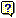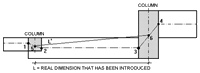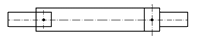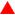Drawings. Beam spans (Real dimension – Drawing dimension) in floor drawings In many occasions, the dimension that has been introduced between column axes does not coincide with the dimension displayed in the drawings. There is a simple explanation for this. The figure displays two columns withdifferent sections to which beams, with different widths, have been adjusted. Upon defining the columns, these were introduced with a distance L between them. The span of the central beam in such an extreme case such as this one is as follows. Points 1, 2, 3 and 4 are cut-off points of the beam axis with the column faces. The mid points are calculated and points 5 and 6 are obtained: distance L, which is the connection of 5 and 6, is thespan of the beam. Hence the span of the beam is greater than the dimension that was introduced. For these cases, when the length to width ratio of the column is large, or a large separation exists between the axis of the column and axis of the beam due to the beam having been adjusted, it may be convenient to divide the alignment so the reinforcement does not consist of continuous bars. The result would be that shown in the following figure. If no beam adjustments have been made, the dimension that was introduced will coincide with the dimension in thebeam drawing. Care should be taken when these cases arise, as the dimension differences can be large.
Top